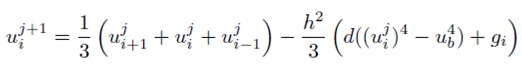#### Finite Difference Method-Nonlinear ODE

Finite Difference Method – Nonlinear ODE:

Heat conduction with radiation:

If we once more consider the heat in a metal bar of length L but this time consider the effect of radiation as well as conduction then the steady state equation has the form

uxx− d(u4− u4b) = −g(x),

Where ub is temperature of the background d incorporates a coefficient of radiation as well as g(x) is the heat source.

If we again restore the continuous problem by its discrete approximation then we get:

(ui+1− 2ui+ ui−1)/ h2− d(u4i− u4b) = −gi= −g(xi).

This equation is nonlinear in the unknowns therefore we no longer have a system of linear equations to solve however a system of nonlinear equations. One way to resolve these equations would be by the multivariable Newton method. In its place we introduce another interactive method.

Relaxation Method for Nonlinear Finite Differences:

We are able to rewrite equation as:

ui+1− 2ui+ ui−1 = h2d(u4i− u4b) − h2gi.

From this we are able to solve for uiin terms of the other quantities:

2ui= ui+1 + ui−1− h2d(u4i− u4b) + h2gi.

After that we add uito both sides of the equation to obtain:

3ui= ui+1 + ui+ ui−1− h2d(u4i− u4b) + h2gi,

And afterwards divide by 3 to get:

ui=1/3(ui+1 + ui+ ui−1) −h2/3(d(u4i− u4b) + gi)

Now for the major idea. We will start with an initial guess for the value of uifor each i which we can represent as a vector u0. Subsequently we will use the above equation to get better estimates, u1, u2, . . . , and expect that they converge to the correct answer.

If we let:

uj= (uj0, uj1, uj2, . . . , ujn−1, ujn)

Denote the jth approximation afterwards we can obtain that j + 1st estimate from the formula:Notice that gi and ub don’t change. In the resulting equation we have ui at every successive step depending on its previous value as well as the equation itself.

Implementing the Relaxation Method:

In the following program we resolve the finite difference equations with the boundary conditions:

u(0) = 0 and u(L) = 0. We let L = 4, n = 4, d = 1, and g(x) = sin(πx/4).

Notice that the vector u forever contains the current estimate of the values of u.

% mynonlinheat (lacks comments)
L = 4; n = 4;
h = L/n; hh = h^2/3;
u0 = 0; uL = 0; ub = .5; ub4 = ub^4;
x = 0:h:L; g = sin(pi*x/4); u = zeros(1,n+1);
steps = 4;
u(1)=u0; u(n+1)=uL;
for j = 1:steps
u(2:n) = (u(3:n+1)+u(2:n)+u(1:n-1))/3 + hh*(-u(2:n).^4+ub4+g(2:n));
end
plot(x,u)

If you run this program with the specified n and steps the result will not seem reasonable.

We are able to plot the initial guess by adding the command plot(x,u) right before the for loop. We can as well plot successive iterations by moving the last plot(x,u) before the end. Now we can experiment as well as see if the iteration is converging. Try a variety of values of steps and n to produce a good plot. You will notice so as to this method converges quite slowly. In particular as we raise n we need to increase steps like n2 that is if n is large then steps needs to be really large.

Latest technology based Matlab Programming Online Tutoring Assistance

Tutors, at the www.tutorsglobe.com, take pledge to provide full satisfaction and assurance in Matlab Programming help via online tutoring. Students are getting 100% satisfaction by online tutors across the globe. Here you can get homework help for Matlab Programming, project ideas and tutorials. We provide email based Matlab Programming help. You can join us to ask queries 24x7 with live, experienced and qualified online tutors specialized in Matlab Programming. Through Online Tutoring, you would be able to complete your homework or assignments at your home. Tutors at the TutorsGlobe are committed to provide the best quality online tutoring assistance for Matlab Programming Homework help and assignment help services. They use their experience, as they have solved thousands of the Matlab Programming assignments, which may help you to solve your complex issues of Matlab Programming. TutorsGlobe assure for the best quality compliance to your homework. Compromise with quality is not in our dictionary. If we feel that we are not able to provide the homework help as per the deadline or given instruction by the student, we refund the money of the student without any delay.